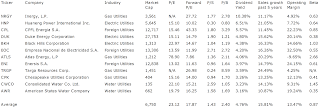## Pages

### 12 Fast Growing Utilities With A Dividend Yield Above 3 Percent

Fast Growing Utility Stocks With Best Dividends by Dividend Yield - Stock, Capital, Investment. Here is a current sheet stocks from the utility industry with a dividend yield of more than 3 percent as well as a sales growth for the past 5 years of more than 10 percent. 12 companies fulfilled these criteria of which 3 are high yields.

Here are the 3 top dividend stocks by dividend yield:

Inergy (NYSE:NRGY) has a market capitalization of \$3.56 billion. The company employs 2,997 people, generates revenues of \$1,786.00 million and has a net income of \$48.70 million. The firm’s earnings before interest, taxes, depreciation and amortization (EBITDA) amounts to \$299.60 million. Because of these figures, the EBITDA margin is 16.77 percent (operating margin 7.72 percent and the net profit margin finally 2.73 percent).

The total debt representing 53.80 percent of the company’s assets and the total debt in relation to the equity amounts to 140.10 percent. Due to the financial situation, the return on equity amounts to -2.39 percent. Finally, earnings per share amounted to \$-0.34 of which \$2.79 were paid in form of dividends to shareholders.

Here are the price ratios of the company: The P/E ratio is not calculable, Price/Sales 2.08 and Price/Book ratio 1.14. Dividend Yield: 9.95 percent. The beta ratio is 0.63.Long-Term Stock Chart Of Inergy, L.P. (Click to enlarge)

Huaneng Power (NYSE:HNP) has a market capitalization of \$6.58 billion. The company employs 33,459 people, generates revenues of \$16,391.89 million and has a net income of \$522.65 million. The firm’s earnings before interest, taxes, depreciation and amortization (EBITDA) amounts to \$3,031.92 million. Because of these figures, the EBITDA margin is 18.50 percent (operating margin 8.28 percent and the net profit margin finally 3.19 percent).

The total debt representing 62.26 percent of the company’s assets and the total debt in relation to the equity amounts to 263.84 percent. Due to the financial situation, the return on equity amounts to 6.98 percent. Finally, earnings per share amounted to \$1.24 of which \$1.26 were paid in form of dividends to shareholders.

Here are the price ratios of the company: The P/E ratio is 15.08, Price/Sales 0.58 and Price/Book ratio 0.74. Dividend Yield: 6.85 percent. The beta ratio is 0.64.Long-Term Stock Chart Of Huaneng Power Intl., I... (Click to enlarge)

CPFL Energia (NYSE:CPL) has a market capitalization of \$12.72 billion. The company employs 8,003 people, generates revenues of \$6,928.11 million and has a net income of \$898.90 million. The firm’s earnings before interest, taxes, depreciation and amortization (EBITDA) amounts to \$1,977.01 million. Because of these figures, the EBITDA margin is 28.54 percent (operating margin 22.78 percent and the net profit margin finally 12.97 percent).

The total debt representing 45.96 percent of the company’s assets and the total debt in relation to the equity amounts to 141.97 percent. Due to the financial situation, the return on equity amounts to 24.11 percent. Finally, earnings per share amounted to \$1.71 of which \$1.51 were paid in form of dividends to shareholders.

Here are the price ratios of the company: The P/E ratio is 15.41, Price/Sales 1.71 and Price/Book ratio 3.30. Dividend Yield: 5.73 percent. The beta ratio is 0.85.Long-Term Stock Chart Of CPFL Energia S.A. (ADR) (Click to enlarge)

Take a closer look at the full table. The average price to earnings ratio (P/E ratio) amounts to 23.1 while the forward P/E ratio is 17.9. The dividend yield has a value of 4.8 percent. Price to book ratio is 2.4 and price to sales ratio 1.4. The operating margin amounts to 13.5 percent.

Here is the table with some fundamentals (TTM):12 Fast Growing Utilities With A Dividend Yield Above 3 Percent (Click to enlarge)

Related stock ticker symbols:
NRGY, HNP, CPL, DUK, BKH, EOC, ATLS, ENI, TRGP, CPK, CWCO, AWR

Selected Articles: